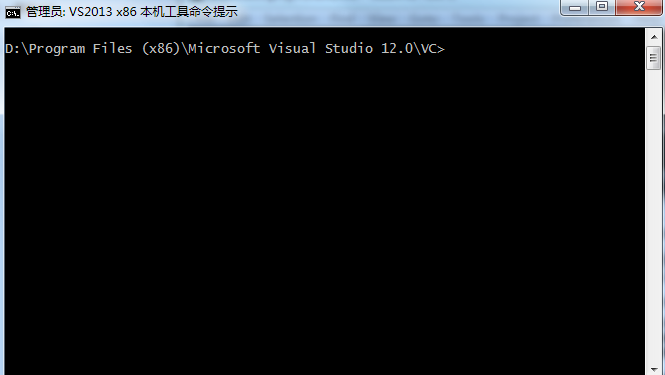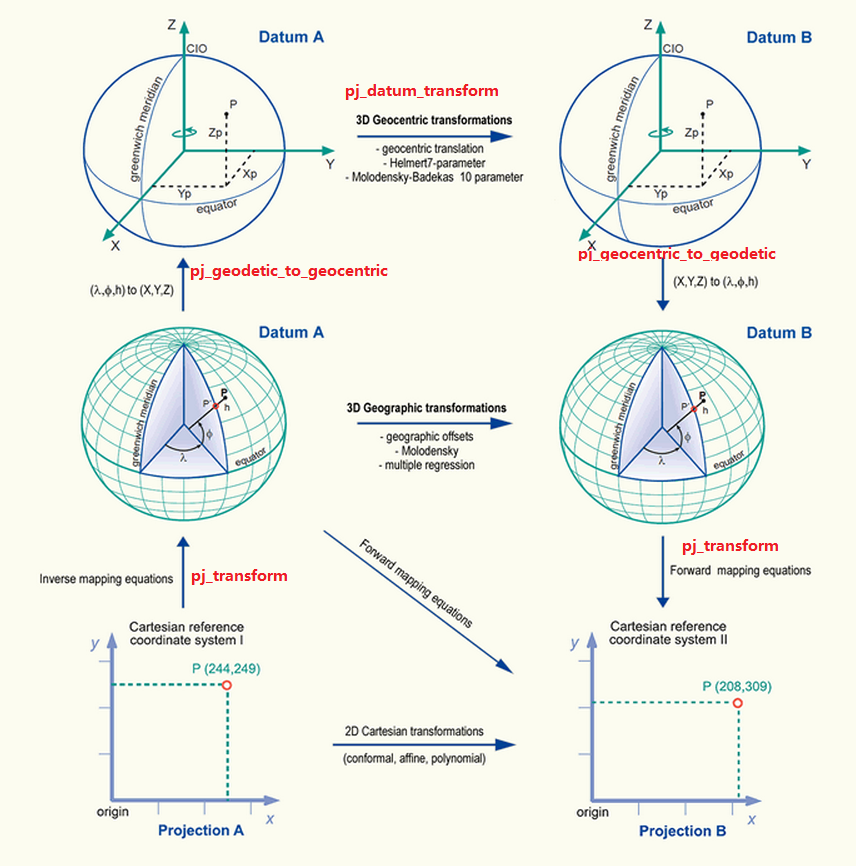# GIS坐标转换库Proj.4的使用

GIS(Geographic Information System 地理信息系统）领域中最常提及 的一个概念是坐标系统，当我们提及一个地理位置的时候，与之伴随而产生的是该位置必定在一个空间参考下。当我们使用GPS设备获取到某个位置的经纬度的时候，我们实际上得到的是一个WGS-84椭球坐标系统下的空间地理坐标。目前空间坐标系统已经十分繁多，这些坐标系统有着特定的使用场合，并且可以通过一定的方式实现它们之间的相互转换，本文提到的Proj.4库正是这样一个提供了不同坐标系统（空间参考）之间相互转换的强大的工具。关于空间参考的详细介绍以及它们之间的转换方法可以参考以下的内容：Geometric
Aspects of Mappin
g

• Proj.4库的安装2013来进行编译）
1. 首先在开始菜单中找到Visual Studio 2013的命令行工具，并启动它：2. 运行下面的命令
``````C:\> cd proj   //进入Proj.4源码目录（以自己的位置为准）
C:\PROJ> nmake /f makefile.vc
C:\PROJ> nmake /f makefile.vc install-all``````

3.由于使用的是默认的命令，因此最终编译的结果会出现在C盘根目录下的PROJ目录下，目录结构如下：• Proj.4工具的使用

1. cs2cs.exe
2. geod.exe
4. proj.exe

• cs2cs.exe
cs2cs工具可以实现两个坐标系统之间的互相转换，这两个坐标系统可以是投影坐标与地理坐标之间的转换，也可以是基准面之间的转换，cs2cs的参数格式如下：
cs2cs [-eEfIlrstvwW [args] ] [+opts[=args] ] [ +to [+opts [=arg] ] ] file[s]
• proj.exe
proj工具用来转换地理坐标到投影坐标以及投影坐标到地理坐标，但是有一个前提条件是这两个坐标系统在同一个基准面之下。 参考：http://geoffair.net/projects/proj4.htm
• geod.exe
geod工具可以计算大圆的距离
• 使用Proj.4库

• 相同基准面之间的转换：投影坐标到地理坐标（经纬度）的转换以及反向转换（从地理坐标到投影坐标之间的转换）

``````#include <proj_api.h>
#include <iostream>

int main(int argc, char **argv)
{
projPJ pj_merc, pj_latlong;
double x, y;

if (!(pj_merc = pj_init_plus("+proj=merc +lon_0=0 +k=1 +x_0=0 +y_0=0 +ellps=WGS84 +datum=WGS84 +units=m +no_defs")))
exit(1);
if (!(pj_latlong = pj_init_plus("+proj=longlat +datum=WGS84 +no_defs")))
exit(1);

x = -9.866554;
y = 7.454779;

pj_transform(pj_latlong, pj_merc, 1, 1, &x, &y, NULL);

std::cout.precision(12);
std::cout << "(" << x << " , " << y << ")" << std::endl;
exit(0);
}``````

``````int pj_transform( projPJ src, projPJ dst, long point_count, int point_offset,
double *x, double *y, double *z );``````

``````#include <proj_api.h>
#include <iostream>

//(-1098339.76716 , 826673.618947)
//	x = -9.866554;
//y = 7.454779;

int main(int argc, char **argv)
{
projPJ pj_merc, pj_latlong;
double x, y;

if (!(pj_merc = pj_init_plus("+proj=merc +lon_0=0 +k=1 +x_0=0 +y_0=0 +ellps=WGS84 +datum=WGS84 +units=m +no_defs")))
exit(1);
if (!(pj_latlong = pj_init_plus("+proj=longlat +datum=WGS84 +no_defs")))
exit(1);

x = -1098339.76716;
y = 826673.618947;

pj_transform(pj_merc, pj_latlong, 1, 1, &x, &y, NULL);

std::cout.precision(12);
std::cout << "(" << x /DEG_TO_RAD<< " , " << y/DEG_TO_RAD << ")" << std::endl;
exit(0);
}``````
• 基准面之间的变换

1. 使用地心坐标系统进行变换，这就要求首先必须将地理坐标转换为地心坐标，由于地心坐标是三维的直角坐标系统，这样两个直角坐标之间的变换就可以使用3参数或者7参数进行变换
2. 直接使用地理坐标进行基准面变换，这种变换方式有相应的算法实现，目前这种方式据我个人目前所知，在Proj4中并没有给出实现

``````int pj_datum_transform( projPJ src, projPJ dst, long point_count, int point_offset,
double *x, double *y, double *z );``````

x, y, z的输入和输出均是 BLH(经纬高程)，src和dst的参数必须包含基准面转换的参数（+towgs84)：可以是三参数或者七参数，类似于：

``````+towgs84=-199.87,74.79,246.62
+towgs=0,0,4.5,0,0,0.554,0.219``````
• 球面坐标到地心坐标的转换以及反转换

``````int pj_geocentric_to_geodetic( double a, double es,
long point_count, int point_offset,
double *x, double *y, double *z );
int pj_geodetic_to_geocentric( double a, double es,
long point_count, int point_offset,
double *x, double *y, double *z );``````